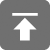# 工学1号馆

home

## 交换两个变量值的泛型实现（C语言）

Wu Yudong    August 27, 2016     C   539

T a = ...;
T b = ...;
.
T tmp = a; a = b; a = tmp;

T a = ...;
T b = ...;
.
T tmpa = a; T tmpb = b;
a = tmpb; b = tmpa;

#define P99_SWAP(A, B) (sizeof(A) > sizeof(uintmax_t) ? P99_SWAP1(A, B) : P99_SWAP2(A, B))

inline
void p00_swap2(void* a, void* b, void* tmpa, void* tmpb, size_t len) {
memcpy(tmpa, a, len);
memcpy(tmpb, b, len);
memcpy(b, tmpa, len);
memcpy(a, tmpb, len);
}
#define P00X_SWAP2(A, B) p00_swap2(
&(A),                                     \
&(B),                                     \
(char[sizeof(A)]){  = 0 },             \
(char[sizeof(A)]){  = 0 }, sizeof(A))

(char[sizeof(A)]){ [(intmax_t)sizeof(A) - sizeof(B)] = 0 }

#define P00_SWAP2(A, B)
p00_swap2(                                                      \
&(A),                                                       \
&(B),                                                       \
(char[sizeof(A)]){ [(intmax_t)sizeof(A) - sizeof(B)] = 0 }, \
(char[sizeof(B)]){ [(intmax_t)sizeof(B) - sizeof(A)] = 0 }, \
sizeof(A))

(1 ? &(A) : ((A = B), NULL))
#define P99_SWAP(A, B) (sizeof(A) > sizeof(uintmax_t) ? P99_SWAP1(A, B) : P99_SWAP2(A, B))